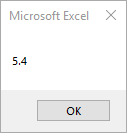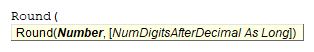# VBA Round Function

## Round Function

Returns a number rounded to a specified number of digits.

## Simple Round Examples

``````Sub Round_Example()
MsgBox Round(5.36, 1)
End Sub
``````

This will return 5.4## Round Syntax

In the VBA Editor, you can type  “Round(” to see the syntax for the Round Function:The Round function contains 2 arguments:

Number: Any valid numeric expression.

NumDigitsAfterDecimal: [Optional] Number indicating how many places to the right of the decimal are included in the rounding (0 if ommitted)

## Examples of Excel VBA Round Function

``MsgBox Round(2.47)``

Result: 2

``MsgBox Round(2.47, 1)``

Result: 2.5

``MsgBox Round(2.5456)``

Result: 3

``MsgBox Round(2.5456, 1)``

Result: 2.5

``MsgBox Round(2.5456, 3)``

Result: 2.546

Stop searching for VBA code online. Learn more about AutoMacro - A VBA Code Builder that allows beginners to code procedures from scratch with minimal coding knowledge and with many time-saving features for all users!